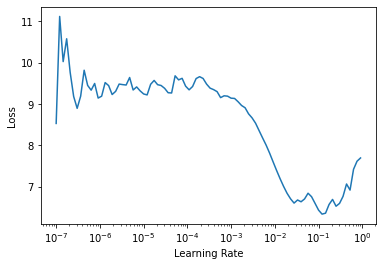Callback and helper functions to schedule any hyper-parameter
from nbdev.showdoc import *

from fastai2.test_utils import *


## Annealing

#### annealer[source]

annealer(f)

Decorator to make f return itself partially applied.

This is the decorator we will use for all of our scheduling functions, as it transforms a function taking (start, end, pos) to something taking (start, end) and return a function depending of pos.

#### sched_lin[source]

sched_lin(start, end, pos)

#### sched_cos[source]

sched_cos(start, end, pos)

#### sched_no[source]

sched_no(start, end, pos)

#### sched_exp[source]

sched_exp(start, end, pos)

annealings = "NO LINEAR COS EXP".split()
p = torch.linspace(0.,1,100)
fns = [SchedNo, SchedLin, SchedCos, SchedExp]

for fn, t in zip(fns, annealings):
plt.plot(p, [fn(2, 1e-2)(o) for o in p], label=t)
f = SchedPoly(2,1e-2,0.5)
plt.plot(p, [f(o) for o in p], label="POLY(0.5)")
plt.legend();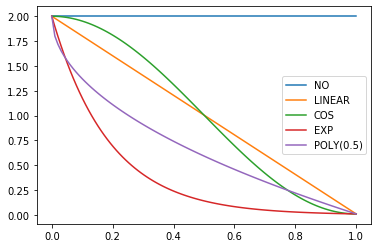#### SchedLin[source]

SchedLin(start, end)

Linear schedule function from start to end

sched = SchedLin(0, 2)
test_eq(L(map(sched, [0., 0.25, 0.5, 0.75, 1.])), [0., 0.5, 1., 1.5, 2.])


#### SchedCos[source]

SchedCos(start, end)

Cosine schedule function from start to end

sched = SchedCos(0, 2)
test_close(L(map(sched, [0., 0.25, 0.5, 0.75, 1.])), [0., 0.29289, 1., 1.70711, 2.])


#### SchedNo[source]

SchedNo(start, end)

Constant schedule function with start value

sched = SchedNo(0, 2)
test_close(L(map(sched, [0., 0.25, 0.5, 0.75, 1.])), [0., 0., 0., 0., 0.])


#### SchedExp[source]

SchedExp(start, end)

Exponential schedule function from start to end

sched = SchedExp(1, 2)
test_close(L(map(sched, [0., 0.25, 0.5, 0.75, 1.])), [1., 1.18921, 1.41421, 1.68179, 2.])


#### SchedPoly[source]

SchedPoly(start, end, power)

Polynomial schedule (of power) function from start to end

sched = SchedPoly(0, 2, 2)
test_close(L(map(sched, [0., 0.25, 0.5, 0.75, 1.])), [0., 0.125, 0.5, 1.125, 2.])

p = torch.linspace(0.,1,100)

pows = [0.5,1.,2.]
for e in pows:
f = SchedPoly(2, 0, e)
plt.plot(p, [f(o) for o in p], label=f'power {e}')
plt.legend();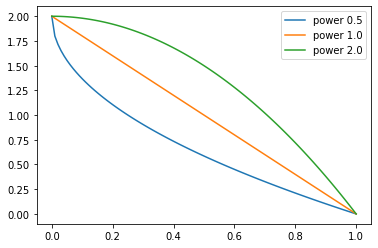#### combine_scheds[source]

combine_scheds(pcts, scheds)

Combine scheds according to pcts in one function

pcts must be a list of positive numbers that add up to 1 and is the same length as scheds. The generated function will use scheds from 0 to pcts then scheds from pcts to pcts+pcts and so forth.

p = torch.linspace(0.,1,100)
f = combine_scheds([0.3,0.7], [SchedCos(0.3,0.6), SchedCos(0.6,0.2)])
plt.plot(p, [f(o) for o in p]);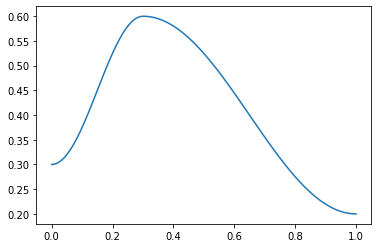p = torch.linspace(0.,1,100)
f = combine_scheds([0.3,0.2,0.5], [SchedLin(0.,1.), SchedNo(1.,1.), SchedCos(1., 0.)])
plt.plot(p, [f(o) for o in p]);


#### combined_cos[source]

combined_cos(pct, start, middle, end)

Return a scheduler with cosine annealing from startmiddle & middleend

This is a useful helper function for the 1cycle policy. pct is used for the start to middle part, 1-pct for the middle to end. Handles floats or collection of floats. For example:

f = combined_cos(0.25,0.5,1.,0.)
plt.plot(p, [f(o) for o in p]);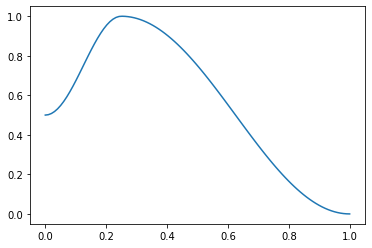## classParamScheduler[source]

ParamScheduler(scheds) :: Callback

Schedule hyper-parameters according to scheds

scheds is a dictionary with one key for each hyper-parameter you want to schedule, with either a scheduler or a list of schedulers as values (in the second case, the list must have the same length as the the number of parameters groups of the optimizer).

learn = synth_learner()
sched = {'lr': SchedLin(1e-3, 1e-2)}
learn.fit(1, cbs=ParamScheduler(sched))
n = len(learn.dls.train)
test_close(learn.recorder.hps['lr'], [1e-3 + (1e-2-1e-3) * i/n for i in range(n)])

(#4) [0,17.4951171875,12.842596054077148,'00:00']


#### ParamScheduler.begin_fit[source]

ParamScheduler.begin_fit()

Initialize container for hyper-parameters

#### ParamScheduler.begin_batch[source]

ParamScheduler.begin_batch()

Set the proper hyper-parameters in the optimizer

#### ParamScheduler.after_batch[source]

ParamScheduler.after_batch()

Record hyper-parameters of this batch

#### ParamScheduler.after_fit[source]

ParamScheduler.after_fit()

Save the hyper-parameters in the recorder if there is one

#### Learner.fit_one_cycle[source]

Learner.fit_one_cycle(n_epoch, lr_max=None, div=25.0, div_final=100000.0, pct_start=0.25, wd=None, moms=None, cbs=None, reset_opt=False)

Fit self.model for n_epoch using the 1cycle policy.

The 1cycle policy was introduced by Leslie N. Smith et al. in Super-Convergence: Very Fast Training of Neural Networks Using Large Learning Rates. It schedules the learning rate with a cosine annealing from lr_max/div to lr_max then lr_max/div_final (pass an array to lr_max if you want to use differential learning rates) and the momentum with cosine annealing according to the values in moms. The first phase takes pct_start of the training. You can optionally pass additional cbs and reset_opt.

learn = synth_learner(lr=1e-2)
xb,yb = learn.dls.one_batch()
init_loss = learn.loss_func(learn.model(xb), yb)
learn.fit_one_cycle(2)
assert learn.loss < init_loss

(#4) [0,11.074447631835938,4.278277397155762,'00:00']
(#4) [1,6.254273891448975,1.2542004585266113,'00:00']

lrs,moms = learn.recorder.hps['lr'],learn.recorder.hps['mom']
test_close(lrs,  [combined_cos(0.25,1e-2/25,1e-2,1e-7)(i/20) for i in range(20)])
test_close(moms, [combined_cos(0.25,0.95,0.85,0.95)(i/20) for i in range(20)])


#### Recorder.plot_sched[source]

Recorder.plot_sched(keys=None, figsize=None)

learn = synth_learner()
learn.fit_one_cycle(2)

(#4) [0,25.833967208862305,20.405487060546875,'00:00']
(#4) [1,23.9661808013916,18.709392547607422,'00:00']

learn.recorder.plot_sched()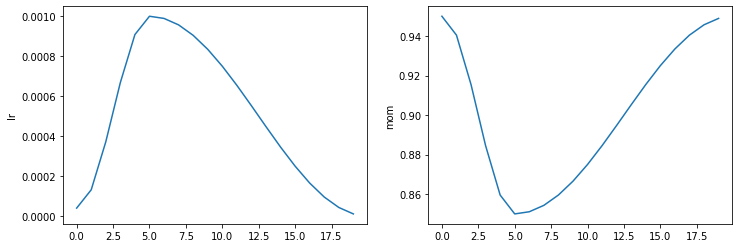#### Learner.fit_flat_cos[source]

Learner.fit_flat_cos(n_epoch, lr=None, div_final=100000.0, pct_start=0.75, wd=None, cbs=None, reset_opt=False)

Fit self.model for n_epoch at flat lr before a cosine annealing.

learn = synth_learner()
learn.fit_flat_cos(2)

(#4) [0,22.340993881225586,23.366474151611328,'00:00']
(#4) [1,19.25973892211914,17.295818328857422,'00:00']

learn.recorder.plot_sched()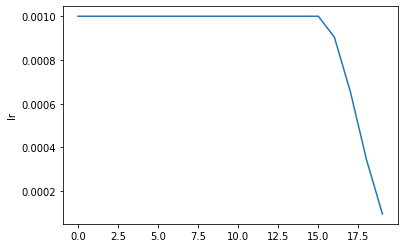#### Learner.fit_sgdr[source]

Learner.fit_sgdr(n_cycles, cycle_len, lr_max=None, cycle_mult=2, cbs=None, reset_opt=False, wd=None)

Fit self.model for n_cycles of cycle_len using SGDR.

This schedule was introduced by Ilya Loshchilov et al. in SGDR: Stochastic Gradient Descent with Warm Restarts. It consists of n_cycles that are cosine annealings from lr_max (defaults to the Learner lr) to 0, with a length of cycle_len * cycle_mult**i for the i-th cycle (first one is cycle_len-long, then we multiply the length by cycle_mult at each epoch). You can optionally pass additional cbs and reset_opt.

learn = synth_learner()
with learn.no_logging(): learn.fit_sgdr(3, 1)
test_eq(learn.n_epoch, 7)
iters = [k * len(learn.dls.train) for k in [0,1,3,7]]
for i in range(3):
n = iters[i+1]-iters[i]
#The start of a cycle can be mixed with the 0 of the previous cycle with rounding errors, so we test at +1
test_close(learn.recorder.lrs[iters[i]+1:iters[i+1]], [SchedCos(learn.lr, 0)(k/n) for k in range(1,n)])

learn.recorder.plot_sched()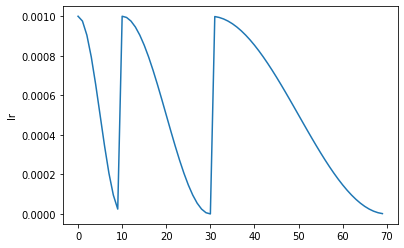#### Learner.fine_tune[source]

Learner.fine_tune(epochs, base_lr=0.002, freeze_epochs=1, lr_mult=100, pct_start=0.3, div=5.0, lr_max=None, div_final=100000.0, wd=None, moms=None, cbs=None, reset_opt=False)

Fine tune with freeze for freeze_epochs then with unfreeze from epochs using discriminative LR

learn.fine_tune(1)

(#4) [0,8.00173282623291,5.4913434982299805,'00:00']
(#4) [0,6.550796031951904,5.056417465209961,'00:00']


## classLRFinder[source]

LRFinder(start_lr=1e-07, end_lr=10, num_it=100, stop_div=True) :: ParamScheduler

Training with exponentially growing learning rate

with tempfile.TemporaryDirectory() as d:
learn = synth_learner(path=Path(d))
init_a,init_b = learn.model.a,learn.model.b
with learn.no_logging(): learn.fit(20, cbs=LRFinder(num_it=100))
assert len(learn.recorder.lrs) <= 100
test_eq(len(learn.recorder.lrs), len(learn.recorder.losses))
#Check stop if diverge
if len(learn.recorder.lrs) < 100: assert learn.recorder.losses[-1] > 4 * min(learn.recorder.losses)
#Test schedule
test_eq(learn.recorder.lrs, [SchedExp(1e-7, 10)(i/100) for i in range_of(learn.recorder.lrs)])
#No validation data
test_eq([len(v) for v in learn.recorder.values], [1 for _ in range_of(learn.recorder.values)])
test_eq(learn.model.a, init_a)
test_eq(learn.model.b, init_b)
test_eq(learn.opt.state_dict()['state'], [{}, {}])


#### LRFinder.begin_fit[source]

LRFinder.begin_fit()

Initialize container for hyper-parameters and save the model

#### LRFinder.begin_batch[source]

LRFinder.begin_batch()

Set the proper hyper-parameters in the optimizer

#### LRFinder.after_batch[source]

LRFinder.after_batch()

Record hyper-parameters of this batch and potentially stop training

#### LRFinder.begin_validate[source]

LRFinder.begin_validate()

Skip the validation part of training

#### Recorder.plot_lr_find[source]

Recorder.plot_lr_find(skip_end=5)

Plot the result of an LR Finder test (won't work if you didn't do learn.lr_find() before)

#### Learner.lr_find[source]

Learner.lr_find(start_lr=1e-07, end_lr=10, num_it=100, stop_div=True, show_plot=True, suggestions=True)

Launch a mock training to find a good learning rate, return lr_min, lr_steep if suggestions is True

First introduced by Leslie N. Smith in Cyclical Learning Rates for Training Neural Networks, the LR Finder trains the model with exponentially growing learning rates from start_lr to end_lr for num_it and stops in case of divergence (unless stop_div=False) then plots the losses vs the learning rates with a log scale.

A good value for the learning rates is then either:

• one tenth of the minimum before the divergence
• when the slope is the steepest

Those two values are returned by default by the Learning Rate Finder.

with tempfile.TemporaryDirectory() as d:
learn = synth_learner(path=Path(d))
lr_min,lr_steep = learn.lr_find()
print(f"Minimum/10: {lr_min:.2e}, steepest point: {lr_steep:.2e}")

Minimum/10: 1.20e-02, steepest point: 7.59e-07